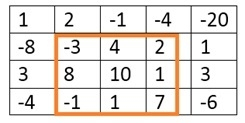# Maximum sum rectangle in a 2D matrix

A matrix is given. We need to find a rectangle (sometimes square) matrix, whose sum is maximum.

The idea behind this algorithm is to fix the left and right columns and try to find the sum of the element from the left column to right column for each row, and store it temporarily. We will try to find top and bottom row numbers. After getting the temporary array, we can apply the Kadane’s Algorithm to get maximum sum sub-array. With it, the total rectangle will be formed.

## Input and Output

Input:
The matrix of integers.
1  2 -1 -4 -20
-8 -3  4  2   1
3  8  10 1   3
-4 -1   1 7  -6

Output:
The top left point and bottom right point of the submatrix, and the total sum of the submatrix.
(Top, Left) (1, 1)
(Bottom, Right) (3, 3)
The max sum is: 29## Algorithm

Input: The array will hold sums, start and end points, number of elements.

Output − Find the starting and ending point.

Begin
sum := 0 and maxSum := - ∞
end := -1
tempStart := 0

for each element i in the array, do
sum := sum + array[i]
if sum < 0, then
sum := 0
tempStart := i + 1
else if sum > maxSum, then
maxSum := sum
start := tempStart
end := i
done

if end ≠ -1, then
return maxSum
maxSum := array, start := 0 and end := 0

for each element i from 1 to n of array, do
if array[i] > maxSum, then
maxSum := array[i]
start := i and end := i
done

return maxSum
End

maxSumRect(Matrix)

Input: The given matrix.

Output: the Maximum sum of the rectangle.

Begin
maxSum := - ∞
define temp array, whose size is same as row of matrix

for left := 0 to number of columns in the Matrix, do
till temp array with 0s
for right := left to column of matrix -1, do
for each row i, do
temp[i] := matrix[i, right]
done

sum := kadaneAlgorithm(temp, start, end, number of rows)
if sum > maxSum, then
maxSum := sum
endLeft := left
endRight := right
endTop := start
endBottom := end
done
done

display top left and bottom right corner and the maxSum
End

## Example

#include<iostream>
#define ROW 4
#define COL 5
using namespace std;

int M[ROW][COL] = {
{1, 2, -1, -4, -20},
{-8, -3, 4, 2, 1},
{3, 8, 10, 1, 3},
{-4, -1, 1, 7, -6}
};

int kadaneAlgo(int arr[], int &start, int &end, int n) {    //find max sum and starting and ending location
int sum = 0, maxSum = INT_MIN;

end = -1;    //at first no place is selected

int tempStart = 0;    //starting from 0

for (int i = 0; i < n; i++) {
sum += arr[i];
if (sum < 0) {
sum = 0;
tempStart = i+1;
}else if (sum > maxSum) {     //get maximum sum, and update start and end index
maxSum = sum;
start = tempStart;
end = i;
}
}

if (end != -1)
return maxSum;
//when all elements are negative in the array
maxSum = arr;
start = end = 0;

// Find the maximum element in array
for (int i = 1; i < n; i++) {
if (arr[i] > maxSum) {
maxSum = arr[i];
start = end = i;
}
}
return maxSum;
}

void maxSumRect() {
int maxSum = INT_MIN, endLeft, endRight, endTop, endBottom;

int left, right;
int temp[ROW], sum, start, end;

for (left = 0; left < COL; left++) {
for(int i = 0; i<ROW; i++)//temp initially holds all 0
temp[i] = 0;

for (right = left; right < COL; ++right) {
for (int i = 0; i < ROW; ++i)    //for each row, find the sum
temp[i] += M[i][right];
sum = kadaneAlgo(temp, start, end, ROW);    //find sum of rectangle (top, left) and (bottom right)

if (sum > maxSum) {    //find maximum value of sum, then update corner points
maxSum = sum;
endLeft = left;
endRight = right;
endTop = start;
endBottom = end;
}
}
}

cout << "(Top, Left) ("<<endTop<<", "<<endLeft<<")"<<endl;
cout << "(Bottom, Right) ("<<endBottom<<", "<<endRight<<")"<<endl;
cout << "The max sum is: "<< maxSum;
}

int main() {
maxSumRect();
}

## Output

(Top, Left) (1, 1)
(Bottom, Right) (3, 3)
The max sum is: 29